﻿ 脉冲输注寨卡的脑癌治疗模型的动力学分析 Dynamic Analysis of the Model of Brain Cancer Treatment by Pulse Infusion of Zika

Vol. 08  No. 03 ( 2019 ), Article ID: 29144 , 16 pages
10.12677/AAM.2019.83050

Dynamic Analysis of the Model of Brain Cancer Treatment by Pulse Infusion of Zika

Yuhong Li, Jian Liu*

School of Mathematics and Information Science, Guangzhou University, Guangzhou Guangdong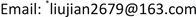Received: Feb. 14th, 2019; accepted: Feb. 27th, 2019; published: Mar. 6th, 2019ABSTRACT

In this paper, we consider a bio-mathematical modeling to construct a pulse infusion of zika virus for the treatment of brain cancer. The boundedness and non-negativeness of the model are obtained. By means of the theory of pulse differential equation, we derive the stability and threshold of the equilibria of the model. Then, the asymptotic stability of the periodic solution under pulse condition is discussed. Finally, the pulse infusion volume of the model is estimated and numerical simulation is carried out.

Keywords:Brain Cancer, Pulse, Stability, Threshold, Periodic Solution1. 模型的提出

2001年，Wodarz在Cancer research上发表了一篇关于病毒缓解肿瘤增长的文章。该文章基于病毒能够感染肿瘤细胞然而对于正常细胞无害这一思想建立了三个常微分方程(ODE)模型  。这些模型分别对应于三种不同的治疗机制，这里仅列举第一个模型。该模型只考虑病毒毒素对肿瘤具有杀灭作用，而关于病毒毒素产生的CTL反应可以清理感染病毒的肿瘤细胞，具体方程如下表示：

$\left\{\begin{array}{l}\frac{\text{d}x}{\text{d}t}=rx\left(1-\frac{x+y}{k}\right)-dx-\beta xy\hfill \\ \frac{\text{d}y}{\text{d}t}=\beta xy+sy\left(1-\frac{x+y}{k}\right)-ay-pyz\hfill \\ \frac{\text{d}z}{\text{d}t}=qyz-bz\hfill \end{array}$ (1)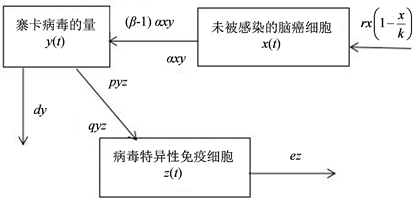Figure 1. Diagram of the relationship between Zika, brain cancer cells and immune response

$\left\{\begin{array}{l}\frac{\text{d}x}{\text{d}t}=rx\left(1-\frac{x}{k}\right)-\alpha xy,\hfill \\ \frac{\text{d}y}{\text{d}t}=\left(\beta -1\right)\alpha xy-dy-pyz,\hfill \\ \frac{\text{d}z}{\text{d}t}=qyz-ez,\hfill \end{array}$ (2)

$\left\{\begin{array}{l}\begin{array}{l}\frac{\text{d}x}{\text{d}t}=rx\left(1-\frac{x}{k}\right)-\alpha xy,\hfill \\ \frac{\text{d}y}{\text{d}t}=\left(\beta -1\right)\alpha xy-dy-pyz\hfill \\ \frac{\text{d}z}{\text{d}t}=qyz-ez,\hfill \end{array},\text{}t\ne nT,\hfill \\ \Delta y\left(t\right)=\stackrel{¯}{y},t=nT,n=1,2,3,\cdots ,\hfill \end{array}$ (3)

2. 没有脉冲输注的情形

${R}_{+}=\left[0,\infty \right)$${R}_{+}^{3}=\left\{\left(x,y,z\right)\in {R}^{3}:x>0,y>0,z>0\right\}$$f=\left({f}_{1},{f}_{2},{f}_{3}\right)$ 表示系统(2)的右端映射， $X:{R}_{+}\to {R}^{3}$ 表示系统(2)的解。显然，系统(2)的解 $X\left(t\right)$${R}_{+}$ 是连续可微的，并且 $f=\left({f}_{1},{f}_{2},{f}_{3}\right)$ 的光滑

$x\left(0\right)>0,y\left(0\right)>0,z\left(0\right)>0.$

$G\left(t\right)=x\left(t\right)+\frac{1}{\beta -1}y\left(t\right)+\frac{p}{q\left(\beta -1\right)}z\left(t\right),\delta =\mathrm{min}\left\{r,d,e\right\}.$

$\frac{\text{d}G\left(t\right)}{\text{d}t}=\frac{\text{d}x}{\text{d}t}+\frac{1}{\beta -1}\frac{\text{d}y}{\text{d}t}+\frac{p}{q\left(\beta -1\right)}\frac{\text{d}z}{\text{d}t}=rx\left(1-\frac{x}{k}\right)-\frac{d}{\beta -1}y-\frac{pe}{q\left(\beta -1\right)}z\le rk-\delta G\left(t\right).$

$\underset{t\to \infty }{\mathrm{lim}}\left[x\left(t\right)+\frac{1}{\beta -1}y\left(t\right)+\frac{p}{q\left(\beta -1\right)}z\left(t\right)\right]\le \frac{rk}{\delta }$ .

$\left\{\begin{array}{l}rx\left(1-\frac{x}{k}\right)-\alpha xy=0,\\ \left(\beta -1\right)\alpha xy-dy-pyz=0,\\ qyz-ez=0.\end{array}$ (4)

${E}_{2}=\left(\frac{d}{\left(\beta -1\right)\alpha },\frac{r}{\alpha }\left[1-\frac{d}{\left(\beta -1\right)k\alpha }\right],0\right)$

${E}_{3}=\left(k\left(1-\frac{e\alpha }{qr}\right),\frac{e}{q},\frac{d}{p}\left[\left(1-\frac{e\alpha }{qr}\right){\Re }_{0}-1\right]\right).$

${R}_{1}=1+\frac{ek\left(\beta -1\right){\alpha }^{2}}{qdr}$ 。下面分情况进行具体分析。

1) 当 ${\Re }_{0}\le 1$ 时，系统(2)存在唯一的原始脑癌平衡点 ${E}_{1}=\left(k,0,0\right)$ ，相应脑癌细胞的浓度达到最大值。此时没有输注寨卡病毒。

2) 当 $1<{\Re }_{0}\le {R}_{1}$ 时，系统(2)存在治疗平衡点 ${E}_{2}$ ，此时，寨卡病毒能够在脑癌细胞内传播，寨卡病毒治疗机制开始产生。

3) 当 ${\Re }_{0}>{R}_{1}$ 时，系统(2)还存在一个内部平衡点——特异性免疫反应平衡点 ${E}_{3}$ ，此时，未感染寨卡的脑癌细胞，寨卡病毒和病毒特异性免疫细胞三者均存在。

$J\left(E\right)=\left(\begin{array}{ccc}r\left(1-\frac{x}{k}\right)-\frac{rx}{k}-\alpha y& -\alpha & 0\\ \left(\beta -1\right)\alpha y& \left(\beta -1\right)\alpha x-d-pz& -py\\ 0& qz& qy-e\end{array}\right).$ (5)

$J\left({E}_{1}\right)=\left(\begin{array}{ccc}-r& -\alpha k& 0\\ 0& \left(\beta -1\right)\alpha k-d& 0\\ 0& 0& -e\end{array}\right).$ (6)

$J\left({E}_{1}\right)$ 的特征方程是，

$\mathrm{det}\left(\lambda I-J\left({E}_{1}\right)\right)=\left(\lambda +r\right)\left(\lambda +e\right)\left[\lambda +d-\left(\beta -1\right)\alpha k\right]=0$ . (7)

${V}_{1}\left(x,y,z\right)=x-{x}_{1}-{x}_{1}\mathrm{ln}\frac{x}{{x}_{1}}+\frac{y}{\beta -1}+\frac{p}{q\left(\beta -1\right)}z.$

$\begin{array}{c}\frac{\text{d}{V}_{1}}{\text{d}t}=\left(1-\frac{{x}_{1}}{x}\right)\frac{\text{d}x}{\text{d}t}+\frac{1}{\beta -1}\frac{\text{d}y}{\text{d}t}+\frac{p}{q\left(\beta -1\right)}\frac{\text{d}z}{\text{d}t}\\ =\left(1-\frac{{x}_{1}}{x}\right)\left[rx\left(1-\frac{x}{k}\right)-\alpha xy\right]+\frac{p}{q\left(\beta -1\right)}\left(qyz-ez\right)+\frac{1}{\beta -1}\left[\left(\beta -1\right)\alpha xy-dy-pyz\right]\\ =\frac{-r\left(x-{x}_{1}\right)\left(x-k\right)}{k}+\alpha {x}_{1}y-\frac{d}{\beta -1}y-\frac{pe}{q\left(\beta -1\right)}z\\ =-\frac{r}{k}{\left(x-k\right)}^{2}+\frac{d}{\beta -1}\left({\Re }_{0}-1\right)y-\frac{pe}{q\left(\beta -1\right)}z\le 0.\end{array}$ (8)

$J\left({E}_{2}\right)=\left(\begin{array}{ccc}\frac{-dr}{k\alpha \left(\beta -1\right)}& \frac{-d}{\beta -1}& 0\\ r\left(\beta -1\right)-\frac{dr}{k\alpha }& 0& \frac{pdr}{k\left(\beta -1\right){\alpha }^{2}}-\frac{pr}{\alpha }\\ 0& 0& \frac{qr}{\alpha }-\frac{qdr}{k\left(\beta -1\right){\alpha }^{2}}-e\end{array}\right).$ (9)

$J\left({E}_{2}\right)$ 的特征方程为，

$\mathrm{det}\left(\lambda I-J\left({E}_{2}\right)\right)=\left(\lambda -\frac{qr}{\alpha }+\frac{qdr}{k\left(\beta -1\right){\alpha }^{2}}+e\right)\left[\lambda \left(\lambda +\frac{dr}{k\alpha \left(\beta -1\right)}\right)+dr-\frac{r{d}^{2}}{k\alpha \left(\beta -1\right)}\right]=0.$

$\left[\lambda -e\left(\frac{qdr}{ek\left(\beta -1\right){\alpha }^{2}}\left({\Re }_{0}-1\right)-1\right)\right]\left[{\lambda }^{2}+\frac{dr\lambda }{k\alpha \left(\beta -1\right)}+\frac{r{d}^{2}}{k\alpha \left(\beta -1\right)}\left({\Re }_{0}-1\right)\right]=0.$ (10)

${V}_{2}\left(x,y,z\right)=x-{x}_{2}-{x}_{2}\mathrm{ln}\frac{x}{{x}_{2}}+\frac{1}{\beta -1}\left[y-{y}_{2}-{y}_{2}\mathrm{ln}\frac{y}{{y}_{2}}\right]+\frac{p}{q\left(\beta -1\right)}z$ .

$\begin{array}{c}\frac{\text{d}{V}_{2}}{\text{d}t}=\left(1-\frac{{x}_{2}}{x}\right)\frac{\text{d}x}{\text{d}t}+\frac{1}{\beta -1}\left(1-\frac{{y}_{2}}{y}\right)\frac{\text{d}y}{\text{d}t}+\frac{p}{q\left(\beta -1\right)}\frac{\text{d}z}{\text{d}t}\\ =\left(x-{x}_{2}\right)\left[r\left(1-\frac{x}{k}\right)-\alpha y\right]+\frac{pz}{q\left(\beta -1\right)}\left(qy-e\right)+\frac{1}{\beta -1}\left(y-{y}_{2}\right)\left[\left(\beta -1\right)\alpha x-d-pz\right]\\ =\left(x-{x}_{2}\right)\left[r-\frac{rx}{k}-\alpha y-\left(r-\frac{r{x}_{2}}{k}-\alpha {y}_{2}\right)\right]+\frac{pz}{q\left(\beta -1\right)}\left[qy-e-\left(q{y}_{2}-e\right)\right]\\ \text{\hspace{0.17em}}\text{\hspace{0.17em}}+\frac{1}{\beta -1}\left(y-{y}_{2}\right)\left\{\left(\beta -1\right)\alpha x-d-pz-\left[\left(\beta -1\right)\alpha {x}_{2}-d-p{z}_{2}\right]\right\}\end{array}$

$\begin{array}{l}=\left(x-{x}_{2}\right)\left[-\frac{r}{k}\left(x-{x}_{2}\right)-\alpha \left(y-{y}_{2}\right)\right]+\frac{pz}{\beta -1}\left(y-{y}_{2}\right)\\ \text{\hspace{0.17em}}\text{\hspace{0.17em}}\text{ }+\frac{1}{\beta -1}\left(y-{y}_{2}\right)\left[\left(\beta -1\right)\alpha \left(x-{x}_{2}\right)-pz\right]\\ =-\frac{r}{k}{\left(x-{x}_{2}\right)}^{2}\le 0\end{array}$ (11)

${V}_{3}\left(x,y,z\right)=x-{x}_{3}-{x}_{3}\mathrm{ln}\frac{x}{{x}_{3}}+\frac{1}{\beta -1}\left[y-{y}_{3}-{y}_{3}\mathrm{ln}\frac{y}{{y}_{3}}\right]+\frac{p}{q\left(\beta -1\right)}\left[z-{z}_{3}-{z}_{3}\mathrm{ln}\frac{z}{{z}_{3}}\right]$ .

$\begin{array}{c}\frac{\text{d}{V}_{3}}{\text{d}t}=\left(1-\frac{{x}_{3}}{x}\right)\frac{\text{d}x}{\text{d}t}+\frac{1}{\beta -1}\left(1-\frac{{y}_{3}}{y}\right)\frac{\text{d}y}{\text{d}t}+\frac{p}{q\left(\beta -1\right)}\left(1-\frac{{z}_{3}}{z}\right)\frac{\text{d}z}{\text{d}t}\\ =\left(x-{x}_{3}\right)\left[r\left(1-\frac{x}{k}\right)-\alpha y\right]+\frac{p}{q\left(\beta -1\right)}\left(qy-e\right)\left(z-{z}_{3}\right)\\ \text{\hspace{0.17em}}\text{\hspace{0.17em}}+\frac{1}{\beta -1}\left(y-{y}_{3}\right)\left[\left(\beta -1\right)\alpha x-d-pz\right]\\ =-\frac{r\left(x-{x}_{3}\right)\left(x-k\right)}{k}-\alpha y\left(x-{x}_{3}\right)+\frac{p}{q\left(\beta -1\right)}\left(q{y}_{3}-e\right)\left(z-{z}_{3}\right)\\ \text{\hspace{0.17em}}\text{\hspace{0.17em}}+\frac{1}{\beta -1}\left(y-{y}_{3}\right)\left[\left(\beta -1\right)\alpha x-d\right]-\frac{p{z}_{3}}{\beta -1}\left(y-{y}_{3}\right)\end{array}$

$\begin{array}{l}=-\frac{r}{k}{\left(x-{x}_{3}\right)}^{2}-\frac{r}{k}\left({x}_{3}-k\right)\left(x-{x}_{3}\right)-\alpha y\left(x-{x}_{3}\right)+\alpha x\left(y-{y}_{3}\right)\\ \text{\hspace{0.17em}}\text{\hspace{0.17em}}\text{ }-\frac{d}{\beta -1}\left(y-{y}_{3}\right)-\frac{p{z}_{3}}{\beta -1}\left(y-{y}_{3}\right)\\ =-\frac{r}{k}{\left(x-{x}_{3}\right)}^{2}+\left(x-{x}_{3}\right)\left[r\left(1-\frac{{x}_{3}}{k}\right)-\alpha {y}_{3}\right]+\left(\alpha {x}_{3}-\frac{d}{\beta -1}\right)\left(y-{y}_{3}\right)-\frac{p{z}_{3}}{\beta -1}\left(y-{y}_{3}\right)\\ =-\frac{r}{k}{\left(x-{x}_{3}\right)}^{2}+0+\frac{y-{y}_{3}}{\beta -1}\left[\left(\beta -1\right)\alpha {x}_{3}-d-p{z}_{3}\right]\\ =-\frac{r}{k}{\left(x-{x}_{3}\right)}^{2}\le 0\end{array}$ (12)

3. 脉冲输注模型的动力学行为

$\left\{\begin{array}{l}\begin{array}{l}\frac{\text{d}x}{\text{d}t}=rx\left(1-\frac{x}{k}\right)-\alpha xy,\hfill \\ \frac{\text{d}y}{\text{d}t}=\left(\beta -1\right)\alpha xy-dy,t\ne nT,\hfill \end{array}\hfill \\ \Delta y\left(t\right)=\stackrel{¯}{y},t=nT,n=1,2,3,\cdots .\hfill \end{array}$ (13)

$\left\{\begin{array}{l}\frac{\text{d}y}{\text{d}t}=-dy,t\ne nT\hfill \\ \Delta y\left(t\right)=y\left({t}^{+}\right)-y\left(t\right)=\stackrel{¯}{y},t=nT\hfill \end{array}$ (14)

$y\left(\left(n+1\right){T}^{+}\right)=f\left(y\left(n{T}^{+}\right)\right)=\left[y\left(n{T}^{+}\right)+\stackrel{¯}{y}\right]{\text{e}}^{-dT}.$

$F:y\left(n{T}^{+}\right)\to y\left(\left(n+1\right){T}^{+}\right)$ 是一个频闪映射，满足方程

$y\left(\left(n+1\right){T}^{+}\right)=f\left(y\left(n{T}^{+}\right)\right)=\left[y\left(n{T}^{+}\right)+\stackrel{¯}{y}\right]{\text{e}}^{-dT}.$

$\stackrel{˜}{y}\left(t\right)={y}^{*}{\text{e}}^{-d\left(t-nT\right)}=\frac{\stackrel{¯}{y}{\text{e}}^{-d\left(t-nT\right)}}{1-{\text{e}}^{-dT}},\left(nT

${E}_{3}=\left(0,\stackrel{˜}{y}\left(t\right),0\right)=\left(0,\frac{\stackrel{¯}{y}{\text{e}}^{-d\left(t-nT\right)}}{1-{e}^{-dT}},0\right),nT

$\left\{\begin{array}{l}\frac{\text{d}X}{\text{d}t}=\left(r-\alpha \stackrel{˜}{y}\left(t\right)\right)X，\\ \frac{\text{d}Y}{\text{d}t}=\left(\beta -1\right)\alpha \stackrel{˜}{y}\left(t\right)X-dY-p\stackrel{˜}{y}\left(t\right)Z,\\ \frac{\text{d}Z}{\text{d}t}=\left(q\stackrel{˜}{y}\left(t\right)-e\right)Z.\end{array}$ (15)

$\stackrel{˜}{y}\left(t\right)={y}^{*}{\text{e}}^{-d\left(t-nT\right)}=\frac{\stackrel{¯}{y}{\text{e}}^{-d\left(t-nT\right)}}{1-{\text{e}}^{-dT}}$$t\in \left(nT,\left(n+1\right)T\right],n\in {Z}_{+}$ ，容易求得：

$X\left(t\right)={x}_{0}\mathrm{exp}\left[{\int }_{0}^{t}\left(r-\alpha \stackrel{˜}{y}\left(s\right)\right)\text{d}s\right]={x}_{0}\mathrm{exp}\left[rt\text{+}\frac{\alpha \stackrel{¯}{y}}{d\left(1-{\text{e}}^{-dT}\right)}\left({\text{e}}^{-dt}-1\right)\right],t\in \left[0,T\right].$

$Z\left(t\right)={z}_{0}\mathrm{exp}\left[{\int }_{0}^{t}\left(q\stackrel{˜}{y}\left(s\right)-e\right)\text{d}s\right]={z}_{0}\mathrm{exp}\left[-\frac{q\stackrel{¯}{y}}{d\left(1-{\text{e}}^{-dT}\right)}\left({\text{e}}^{-dt}-1\right)-et\right],t\in \left[0,T\right].$

$Y\left(t\right)={\text{e}}^{-dt}\left({\int }_{0}^{t}\left[\left(\beta -1\right)\alpha X\left(s\right)-pZ\left(s\right)\right]\stackrel{˜}{y}\left(s\right){\text{e}}^{ds}\text{d}s+{y}_{0}\right).$ (16)

$\left({x}_{0},{y}_{0},{z}_{0}\right)$ 分别为 $\left(1,0,0\right),\left(0,1,0\right),\left(0,0,1\right)$ ，可得解 $\left({X}_{i}\left(t\right),{Y}_{i}\left(t\right),{Z}_{i}\left(t\right)\right),i=1,2,3$

$\Phi \left(t\right)=\left(\begin{array}{ccc}{X}^{*}\left(t\right)& 0& 0\\ P\left(t\right)& {Y}^{*}\left(t\right)& Q\left(t\right)\\ 0& 0& {Z}^{*}\left(t\right)\end{array}\right),t\in \left[0,T\right].$

${X}^{*}\left(t\right)=\mathrm{exp}\left[rt+\frac{\alpha \stackrel{¯}{y}}{d\left(1-{\text{e}}^{-dT}\right)}\left({\text{e}}^{-dt}-1\right)\right],$

${Y}^{*}\left(t\right)={\text{e}}^{-dt},$

${Z}^{*}\left(t\right)=\mathrm{exp}\left[-et+\frac{q\stackrel{¯}{y}}{d\left(1-{\text{e}}^{-dT}\right)}\left(1-{\text{e}}^{-dt}\right)\right],$

$P\left(t\right)=\left(\beta -1\right)\alpha {\text{e}}^{-dt}{\int }_{0}^{t}{X}^{*}\left(s\right)\stackrel{˜}{y}\left(s\right){\text{e}}^{ds}\text{d}s,Q\left(t\right)=-p{\text{e}}^{-dt}{\int }_{0}^{t}{Z}^{*}\left(s\right)\stackrel{˜}{y}\left(s\right){\text{e}}^{ds}\text{d}s.$

$\left(\begin{array}{ccc}{X}_{1}\left(0\right)& {X}_{2}\left(0\right)& {X}_{3}\left(0\right)\\ {Y}_{1}\left(0\right)& {Y}_{2}\left(0\right)& {Y}_{3}\left(0\right)\\ {Z}_{1}\left(0\right)& {Z}_{2}\left(0\right)& {Z}_{3}\left(0\right)\end{array}\right)=\left(\begin{array}{ccc}1& 0& 0\\ 0& 1& 0\\ 0& 0& 1\end{array}\right).$

$M=\left(\begin{array}{ccc}1& 0& 0\\ 0& 1& 0\\ 0& 0& 1\end{array}\right)\Phi \left(T\right)=\Phi \left(T\right)=\left(\begin{array}{ccc}\mathrm{exp}\left[rT-\frac{\alpha \stackrel{¯}{y}}{d}\right]& 0& 0\\ \Delta & {\text{e}}^{-dT}& \Delta \\ 0& 0& \mathrm{exp}\left[-eT+\frac{q\stackrel{¯}{y}}{d}\right]\end{array}\right).$

${\lambda }_{1}=\text{exp}\left[rT-\frac{\alpha \stackrel{¯}{y}}{d}\right],{\lambda }_{3}=\mathrm{exp}\left[-eT+\frac{q\stackrel{¯}{y}}{d}\right],{\lambda }_{2}={\text{e}}^{-dT}.$

4. 脉冲输注量估计$rT+\frac{\alpha \stackrel{¯}{y}}{-d}<0,-eT+\frac{q\stackrel{¯}{y}}{d}<0,$

$\frac{rdT}{\alpha }<\stackrel{¯}{y}<\frac{edT}{q},\left(\frac{r}{\alpha }<\frac{e}{q}\right),\frac{q\stackrel{¯}{y}}{de}

$T\in \left(\frac{q\stackrel{¯}{y}}{de},\frac{\alpha \stackrel{¯}{y}}{rd}\right),\left(\frac{r}{\alpha }<\frac{e}{q}\right)$ 时，系统(3)的T-周期解 ${E}_{3}=\left(0,\stackrel{˜}{y}\left(t\right),0\right)$ 是局部渐近稳定的。

5. 数值模拟、总结Figure 2. Change in solution (2) of the system without pulse effect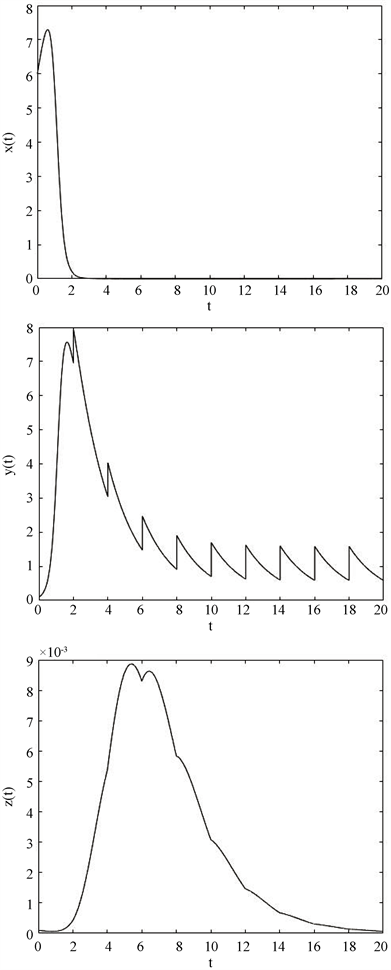Figure 3. When the pulse period and zika infusion amount are respectively $T=2,\stackrel{¯}{y}=1$ , the solution curve of system (2)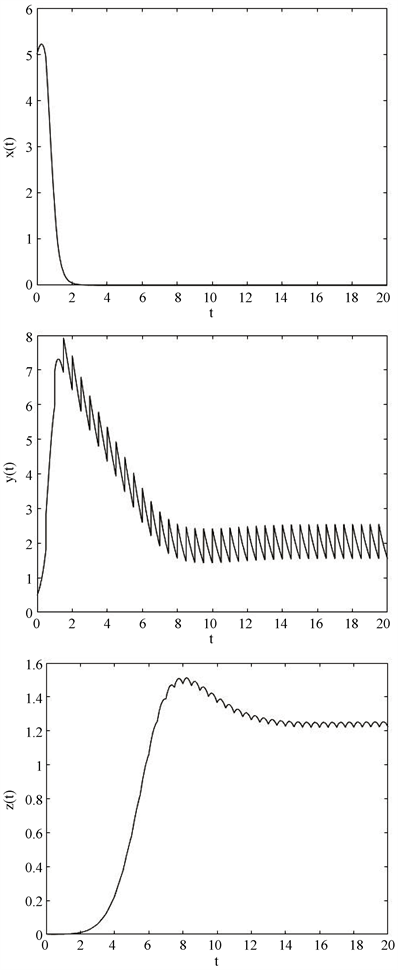Figure 4. When the pulse period and zika infusion amount are respectively $T=0.5,\stackrel{¯}{y}=1$ , the solution curve of system (2)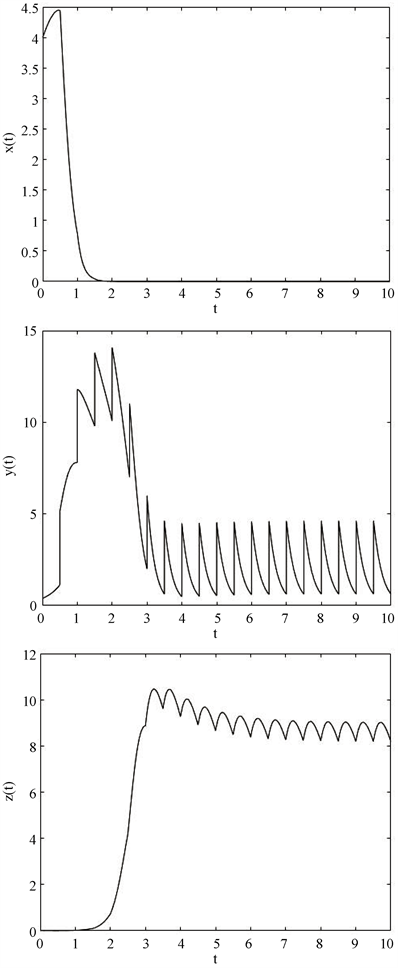Figure 5. When the pulse period and zika infusion amount are respectively $T=0.5,\stackrel{¯}{y}=4$ , the solution curve of system (2)Figure 6. When the pulse period and zika infusion amount are respectively $T=4,\stackrel{¯}{y}=4$ , the solution curve of system (2)

Dynamic Analysis of the Model of Brain Cancer Treatment by Pulse Infusion of Zika[J]. 应用数学进展, 2019, 08(03): 439-454. https://doi.org/10.12677/AAM.2019.83050

1. 1. Tian, J.P. (2011) The Replicability of Oncolytic Virus: Defining Conditions in Tumor Virotherapy. Mathematical Bio-sciences and Engineering, 8, 841-860. https://doi.org/10.3934/mbe.2011.8.841

2. 2. Wodarz, D. (2001) Viruses as Antitumor Weapons. Cancer Research, 61, 3501-3507.

3. 3. Patel, M.R. and Kratzke, R.A. (2013) Oncolytic Virus Therapy for Cancer: The First Wave of Translational Clinical trials. Translational Research, 161, 355-364. https://doi.org/10.1016/j.trsl.2012.12.010

4. 4. Dingli, D., Cascino, M.D., Josic, K., et al. (2006) Mathematical Modeling of Cancer Radiovirotherapy. Mathematical Biosciences, 199, 55-78. https://doi.org/10.1016/j.mbs.2005.11.001

5. 5. 寨卡病毒或可治疗脑癌[J]. 中国肿瘤临床与康复, 2017, 24(11): 1394.

6. 6. Zhu, Z., Gorman, M.J., McKenzie, L.D., et al. (2017) Zika Virus Has Oncolytic Activity against Glioblas-toma Stem Cells. Journal of Experimental Medicine, 214, 2843-2857. https://doi.org/10.1084/jem.20171093

7. 7. Zhou, L. (2013) The Clinical Research Progress for Oncolytic Ade-novirus Targeting Cancer Therapy. China Biotechnology, 33, 105-113.

8. 8. 王子子, 郭志明. 关于肿瘤溶瘤病毒疗法的数学模型及其分析[J]. 广州大学学报(自然科学版), 2017, 16(4): 14-26.

9. 9. LaSalle, J.P., 著, 陆征一, 译. 动力系统的稳定性[M]. 成都: 四川科学技术出版社, 2002.

10. 10. 张玉娟, 陈兰荪, 孙丽华. 一类具有脉冲效应的捕食者–食饵系统分析[J]. 大连理工大学学报, 2004(5): 769-774.

11. 11. 潘玉娜. 脉冲输注免疫因子的HIV治疗模型的动力学性质研究[D]: [硕士学位论文]. 衡阳: 南华大学, 2014.

12. 12. 曾广钊. 两次脉冲的微分方程数值模拟程序的设计[J]. 中山大学学报论丛, 2005(3): 131-133.

13. NOTES

*通讯作者。